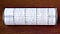A meaningful representation of the input, you must encode

This is Part 1/2 of Dissecting BERT written by Miguel Romero and Francisco Ingham. Each article was written jointly by both authors. If you already understand the Encoder architecture from Attention is All You Need and you are interested in the differences that make BERT awesome, head on to BERT Specifics.

Many thanks to Yannet Interian for her review and feedback.

In this blog post, we are going to examine the Encoder architecture in depth (see Figure 1) as described in Attention Is All You Need. In BERT Specifics we will dive into the novel modifications that make BERT particularly effective.

# Notation

Before we begin, let’s define the notation we will use throughout the article:

emb_dim: Dimension of the token embeddings.

input_length: Length of the input sequence (the same in all sequences in a specific batch due to padding).

hidden_dim: Size of the Feed-Forward network’s hidden layer.

vocab_size: Amount of words in the vocabulary (derived from the corpus).

# Introduction

The Encoder used in BERT is an attention-based architecture for Natural Language Processing (NLP) that was introduced in the paper Attention Is All You Need a year ago. The paper introduces an architecture called the Transformer which is composed of two parts, the Encoder and the Decoder. Since BERT only uses the Encoder we are only going to explain that in this blog post (if you want to learn about the Decoder and how it is integrated with the Encoder, we wrote a separate blog post on this).

Transfer learning has quickly become a standard for state of the art results in NLP since the release of ULMFiT earlier this year. After that, remarkable advances have been achieved by combining the Transformer with transfer learning. Two iconic examples of this combination are OpenAI’s GPT and Google AI’s BERT.

This series aims to:

1. Provide an intuitive understanding of the Transformer and BERT’s underlying architecture.
2. Explain the fundamental principles of what makes BERT so successful in NLP tasks.

To explain this architecture we will adopt the general to specifics approach. We will start by looking at the information flow in the architecture and we will dive into the inputs and outputs of the Encoder as presented in the paper. Next, we will look into each of the encoder blocks and understand how Multi-Head Attention is used. Don't worry if you don't know what that is yet; we will make sure you understand it by the end of this article.

# Information Flow

The data flow through the architecture is as follows:

1. The model represents each token as a vector of emb_dim size. With one embedding vector for each of the input tokens, we have a matrix of dimensions (input_length) x (emb_dim) for a specific input sequence.
2. It then adds positional information (positional encoding). This step returns a matrix of dimensions (input_length) x (emb_dim), just like in the previous step.
3. The data goes through N encoder blocks. After this, we obtain a matrix of dimensions (input_length) x (emb_dim).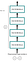Figure 2: Information flow in the Encoder

Note: The dimensions of the input and output of the encoder block are the same. Hence, it makes sense to use the output of one encoder block as the input of the next encoder block.

Note: In BERT's experiments, the number of blocks N (or L, as they call it) was chosen to be 12 and 24.

Note: The blocks do not share weights with each other

# From words to vectors

## Tokenization, numericalization and word embeddings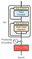Figure 3: Where tokenization, numericalization and embeddings happen.

Tokenization, numericalization and embeddings do not differ from the way it is done with RNNs. Given a sentence in a corpus:

“ Hello, how are you?”

The first step is to tokenize it:

“ Hello, how are you?” → [“Hello”, “,” , “how”, “are”, “you”, “?”]

This is followed by numericalization, mapping each token to a unique integer in the corpus’ vocabulary.

[“Hello”, “, “, “how”, “are”, “you”, “?”] → [34, 90, 15, 684, 55, 193]

Next, we get the embedding for each word in the sequence. Each word of the sequence is mapped to a emb_dim dimensional vector that the model will learn during training. You can think about it as a vector look-up for each token. The elements of those vectors are treated as model parameters and are optimized with back-propagation just like any other weights.

Therefore, for each token, we look up the corresponding vector:

Stacking each of the vectors together we obtain a matrix Z of dimensions (input_length) x (emb_dim):

It is important to remark that padding was used to make the input sequences in a batch have the same length. That is, we increase the length of some of the sequences by adding ‘<pad>’ tokens. The sequence after padding might be:

[“<pad>”, “<pad>”, “<pad>”, “Hello”, “, “, “how”, “are”, “you”, “?”] →

[5, 5, 5, 34, 90, 15, 684, 55, 193]

if the input_length was set to 9.

## Positional Encoding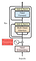Figure 4: Where Positional Encoding is computed.

Note: In BERT the authors used learned positional embeddings. If you are only interested in BERT you can skip this section where we explain the functions used to calculate the positional encodings in Attention is All You Need

At this point, we have a matrix representation of our sequence. However, these representations are not encoding the fact that words appear in different positions.

Intuitively, we aim to be able to modify the represented meaning of a specific word depending on its position. We don't want to change the full representation of the word but we want to modify it a little to encode its position.

The approach chosen in the paper is to add numbers between [-1,1] using predetermined (non-learned) sinusoidal functions to the token embeddings. Observe that now, for the rest of the Encoder, the word will be represented slightly differently depending on the position the word is in (even if it is the same word).

Moreover, we would like the Encoder to be able to use the fact that some words are in a given position while, in the same sequence, other words are in other specific positions. That is, we want the network to able to understand relative positions and not only absolute ones. The sinuosidal functions chosen by the authors allow positions to be represented as linear combinations of each other and thus allow the network to learn relative relationships between the token positions.

The approach chosen in the paper to add this information is adding to Z a matrix P with positional encodings.

Z + P

The authors chose to use a combination of sinusoidal functions. Mathematically, using i for the position of the token in the sequence and j for the position of the embedding feature:

More specifically, for a given sentence P, the positional embedding matrix would be as follows:

The authors explain that the result of using this deterministic method instead of learning positional representations (just like we did with the embeddings) lead to similar performance. Moreover, this approach had some specific advantages over learned positional representations:

• The input_length can be increased indefinitely since the functions can be calculated for any arbitrary position.
• Fewer parameters needed to be learned and the model trained quicker.

The resulting matrix:

X = Z + P

is the input of the first encoder block and has dimensions (input_length) x (emb_dim).

# Encoder block

A total of N encoder blocks are chained together to generate the Encoder’s output. A specific block is in charge of finding relationships between the input representations and encode them in its output.

Intuitively, this iterative process through the blocks will help the neural network capture more complex relationships between words in the input sequence. You can think about it as iteratively building the meaning of the input sequence as a whole.

The Transformer uses Multi-Head Attention, which means it computes attention h different times with different weight matrices and then concatenates the results together.

The result of each of those parallel computations of attention is called a head. We are going to denote a specific head and the associated weight matrices with the subscript i.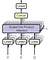Figure 7: Illustration of the parallel heads computations and their concatenation

As shown in Figure 7, once all the heads have been computed they will be concatenated. This will result in a matrix of dimensions (input_length) x (h*d_v). Afterwards, a linear layer with weight matrix W⁰ of dimensions (h*d_v) x (emb_dim) will be applied leading to a final result of dimensions (input_length) x (emb_dim). Mathematically:

Where Q,K and V are placeholders for different input matrices. In particular, for this case Q,K and V will be replaced by the output matrix of the previous step X.

# Scaled Dot-Product Attention

## Overview

Each head is going to be characterized by three different projections (matrix multiplications) given by matrices:

To compute a head we will take the input matrix X and separately project it with the above weight matrices:

Note: In the paper d_k and d_v are set such that d_k = d_v = emb_dim/h

Once we have K_i, Q_i and V_i we use them to compute the Scaled Dot-Product Attention:

Graphically: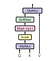Figure 8: Illustration of the Dot-Product Attention.

Note: In the encoder block the computation of attention does not use a mask. In our Decoder post we explain how the decoder uses masking.

## Going Deeper

This is the key of the architecture (the name of the paper is no coincidence) so we need to understand it carefully. Let’s start by looking at the matrix product between Q_i and K_i transposed:

Remember that Q_i and K_i were different projections of the tokens into a d_k dimensional space. Therefore, we can think about the dot product of those projections as a measure of similarity between tokens projections. For every vector projected through Q_i the dot product with the projections through K_i measures the similarity between those vectors. If we call v_i and u_j the projections of the i-th token and the j-th token through Q_i and K_i respectively, their dot product can be seen as:

Thus, this is a measure of how similar are the directions of u_i and v_j and how large are their lengths (the closest the direction and the larger the length, the greater the dot product).

Another way of thinking about this matrix product is as the encoding of a specific relationship between each of the tokens in the input sequence (the relationship is defined by the matrices K_i, Q_i).

After this multiplication, the matrix is divided element-wise by the square root of d_k for scaling purposes.

The next step is a Softmax applied row-wise (one softmax computation for each row):

In our example, this could be:

The result would is rows with numbers between zero and one that sum to one. Finally, the result is multiplied by V_i to get the result of the head.

## Example 1

For the sake of understanding let’s propose a dummy example. Suppose that the resulting first row of:

is [0,0,0,0,1,0]. Hence, because 1 is in the 5th position of the vector, the result will then be:

Where v_{token} is the projection through V_i of the token’s representation. Observe that in this case the word “hello” ends up with a representation based on the 4th token “you” of the input for that head.

Supposing an equivalent example for the rest of the heads. The word “Hello” will be now represented by the concatenation of the different projections of other words. The network will learn over training time which relationships are more useful and will relate tokens to each other based on these relationships.

## Example 2

Let us now complicate the example a little bit more. Suppose now our previous example in the more general scenario where there isn’t just a single 1 per row but decimal positive numbers that sum to 1:

If we do as in the previous example and multiply that by V_i:

This results in a matrix where each row is a composition of the projection of the token’s representations through V_i:

Observe that we can think about the resulting representation of “Hello” as a weighted combination (centroid) of the projected vectors through V_i of the input tokens.

Thus, a specific head captures a specific relationship between the input tokens. Now, if we do that h times (a total of h heads) each encoder block is capturing h different relationships between input tokens.

Following up, assume that the example above referred to the first head. Then the first row would be:

Then the first row of the result of the Multi-Head Attention layer, i.e. the representation of “Hello” at this point, would be

Which is a vector of length emb_dim given that the matrix W_0 has dimensions (d_v*h) x (emb_dim). Applying the same logic in the rest of the rows/tokens representations we obtain a matrix of dimensions (input_length) x (emb_dim).

Thus, at this point, the representation of the token is the concatenation of h weighted combinations of token representations (centroids) through the h different learned projections.

# Position-wise Feed-Forward Network

This step is composed of the following layers: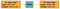Figure 10: Scheme of the Feed Forwards Neural Netwrok

Mathematically, for each row in the output of the previous layer:

where W_1 and W_2 are (emb_dim) x (d_F) and (d_F) x (emb_dim) matrices respectively.

Observe that during this step, vector representations of tokens don’t “interact” with each other. It is equivalent to run the calculations row-wise and stack the resulting rows in a matrix.

The output of this step has dimension (input_length) x (emb_dim).

# Dropout, Add & Norm

Before this layer, there is always a layer for which inputs and outputs have the same dimensions (Multi-Head Attention or Feed-Forward). We will call that layer Sublayer and its input x.

After each Sublayer, dropout is applied with 10% probability. Call this result Dropout(Sublayer(x)). This result is added to the Sublayer’s input x, and we get x + Dropout(Sublayer(x)).

Observe that in the context of a Multi-Head Attention layer, this means adding the original representation of a token x to the representation based on the relationship with other tokens. It is like telling the token:

“Learn the relationship with the rest of the tokens, but don’t forget what we already learned about yourself!”

Finally, a token-wise/row-wise normalization is computed with the mean and standard deviation of each row. This improves the stability of the network.

The output of these layers is:

And that’s it! This is the architecture behind all of the magic in state of the art NLP.

If you have any feedback please let us know in the comment section!

## References

Attention Is All You Need; Vaswani et al., 2017.

BERT: Pre-training of Deep Bidirectional Transformers for Language Understanding; Devlin et al., 2018.

The Annotated Transformer; Alexander Rush, Vincent Nguyen and Guillaume Klein.

Universal Language Model Fine-tuning for Text Classification; Howard et al., 2018.

Improving Language Understanding by Generative Pre-Training; Radford et al., 2018.

Source of cover picture: Cripttografia e numeri primi

Written by

## Dissecting BERT

#### Understand BERT in depth with an intuitive, straightforward explanation of the relevant concepts

Welcome to a place where words matter. On Medium, smart voices and original ideas take center stage - with no ads in sight. Watch
Follow all the topics you care about, and we’ll deliver the best stories for you to your homepage and inbox. Explore
Get unlimited access to the best stories on Medium — and support writers while you’re at it. Just \$5/month. Upgrade